# 6. OOP I: Objects and Names¶

## 6.1. Overview¶

Object-oriented programming (OOP) is one of the major paradigms in programming.

The traditional programming paradigm (think Fortran, C, MATLAB, etc.) is called procedural.

It works as follows

• The program has a state corresponding to the values of its variables.

• Functions are called to act on these data.

• Data are passed back and forth via function calls.

In contrast, in the OOP paradigm

• data and functions are “bundled together” into “objects”

(Functions in this context are referred to as methods)

### 6.1.1. Python and OOP¶

Python is a pragmatic language that blends object-oriented and procedural styles, rather than taking a purist approach.

However, at a foundational level, Python is object-oriented.

In particular, in Python, everything is an object.

In this lecture, we explain what that statement means and why it matters.

## 6.2. Objects¶

In Python, an object is a collection of data and instructions held in computer memory that consists of

1. a type

2. a unique identity

3. data (i.e., content)

4. methods

These concepts are defined and discussed sequentially below.

### 6.2.1. Type¶

Python provides for different types of objects, to accommodate different categories of data.

For example

s = 'This is a string'
type(s)

str

x = 42   # Now let's create an integer
type(x)

int


The type of an object matters for many expressions.

For example, the addition operator between two strings means concatenation

'300' + 'cc'

'300cc'


On the other hand, between two numbers it means ordinary addition

300 + 400

700


Consider the following expression

'300' + 400

---------------------------------------------------------------------------
TypeError                                 Traceback (most recent call last)
/tmp/ipykernel_1098/1595573990.py in <module>
----> 1 '300' + 400

TypeError: can only concatenate str (not "int") to str


Here we are mixing types, and it’s unclear to Python whether the user wants to

• convert '300' to an integer and then add it to 400, or

• convert 400 to string and then concatenate it with '300'

Some languages might try to guess but Python is strongly typed

• Type is important, and implicit type conversion is rare.

• Python will respond instead by raising a TypeError.

To avoid the error, you need to clarify by changing the relevant type.

For example,

int('300') + 400   # To add as numbers, change the string to an integer

700


### 6.2.2. Identity¶

In Python, each object has a unique identifier, which helps Python (and us) keep track of the object.

The identity of an object can be obtained via the id() function

y = 2.5
z = 2.5
id(y)

139866423043728

id(z)

139866423042960


In this example, y and z happen to have the same value (i.e., 2.5), but they are not the same object.

The identity of an object is in fact just the address of the object in memory.

### 6.2.3. Object Content: Data and Attributes¶

If we set x = 42 then we create an object of type int that contains the data 42.

In fact, it contains more, as the following example shows

x = 42
x

42

x.imag

0

x.__class__

int


When Python creates this integer object, it stores with it various auxiliary information, such as the imaginary part, and the type.

Any name following a dot is called an attribute of the object to the left of the dot.

• e.g.,imag and __class__ are attributes of x.

We see from this example that objects have attributes that contain auxiliary information.

They also have attributes that act like functions, called methods.

These attributes are important, so let’s discuss them in-depth.

### 6.2.4. Methods¶

Methods are functions that are bundled with objects.

Formally, methods are attributes of objects that are callable (i.e., can be called as functions)

x = ['foo', 'bar']
callable(x.append)

True

callable(x.__doc__)

False


Methods typically act on the data contained in the object they belong to, or combine that data with other data

x = ['a', 'b']
x.append('c')
s = 'This is a string'
s.upper()

'THIS IS A STRING'

s.lower()

'this is a string'

s.replace('This', 'That')

'That is a string'


A great deal of Python functionality is organized around method calls.

For example, consider the following piece of code

x = ['a', 'b']
x = 'aa'  # Item assignment using square bracket notation
x

['aa', 'b']


It doesn’t look like there are any methods used here, but in fact the square bracket assignment notation is just a convenient interface to a method call.

What actually happens is that Python calls the __setitem__ method, as follows

x = ['a', 'b']
x.__setitem__(0, 'aa')  # Equivalent to x = 'aa'
x

['aa', 'b']


(If you wanted to you could modify the __setitem__ method, so that square bracket assignment does something totally different)

## 6.3. Names and Name Resolution¶

### 6.3.1. Variable Names in Python¶

Consider the Python statement

x = 42


We now know that when this statement is executed, Python creates an object of type int in your computer’s memory, containing

• the value 42

• some associated attributes

But what is x itself?

In Python, x is called a name, and the statement x = 42 binds the name x to the integer object we have just discussed.

Under the hood, this process of binding names to objects is implemented as a dictionary—more about this in a moment.

There is no problem binding two or more names to the one object, regardless of what that object is

def f(string):      # Create a function called f
print(string)   # that prints any string it's passed

g = f
id(g) == id(f)

True

g('test')

test


In the first step, a function object is created, and the name f is bound to it.

After binding the name g to the same object, we can use it anywhere we would use f.

What happens when the number of names bound to an object goes to zero?

Here’s an example of this situation, where the name x is first bound to one object and then rebound to another

x = 'foo'
id(x)

139866482880240

x = 'bar'  # No names bound to the first object


What happens here is that the first object is garbage collected.

In other words, the memory slot that stores that object is deallocated, and returned to the operating system.

Garbage collection is actually an active research area in computer science.

You can read more on garbage collection if you are interested.

### 6.3.2. Namespaces¶

Recall from the preceding discussion that the statement

x = 42


binds the name x to the integer object on the right-hand side.

We also mentioned that this process of binding x to the correct object is implemented as a dictionary.

This dictionary is called a namespace.

Definition: A namespace is a symbol table that maps names to objects in memory.

Python uses multiple namespaces, creating them on the fly as necessary.

For example, every time we import a module, Python creates a namespace for that module.

To see this in action, suppose we write a script mathfoo.py with a single line

%%file mathfoo.py
pi = 'foobar'

Writing mathfoo.py


Now we start the Python interpreter and import it

import mathfoo


Next let’s import the math module from the standard library

import math


Both of these modules have an attribute called pi

math.pi

3.141592653589793

mathfoo.pi

'foobar'


These two different bindings of pi exist in different namespaces, each one implemented as a dictionary.

We can look at the dictionary directly, using module_name.__dict__

import math

math.__dict__.items()

dict_items([('__name__', 'math'), ('__doc__', 'This module provides access to the mathematical functions\ndefined by the C standard.'), ('__package__', ''), ('__loader__', <_frozen_importlib_external.ExtensionFileLoader object at 0x7f35352a22e0>), ('__spec__', ModuleSpec(name='math', loader=<_frozen_importlib_external.ExtensionFileLoader object at 0x7f35352a22e0>, origin='/opt/conda/envs/quantecon/lib/python3.9/lib-dynload/math.cpython-39-x86_64-linux-gnu.so')), ('acos', <built-in function acos>), ('acosh', <built-in function acosh>), ('asin', <built-in function asin>), ('asinh', <built-in function asinh>), ('atan', <built-in function atan>), ('atan2', <built-in function atan2>), ('atanh', <built-in function atanh>), ('ceil', <built-in function ceil>), ('copysign', <built-in function copysign>), ('cos', <built-in function cos>), ('cosh', <built-in function cosh>), ('degrees', <built-in function degrees>), ('dist', <built-in function dist>), ('erf', <built-in function erf>), ('erfc', <built-in function erfc>), ('exp', <built-in function exp>), ('expm1', <built-in function expm1>), ('fabs', <built-in function fabs>), ('factorial', <built-in function factorial>), ('floor', <built-in function floor>), ('fmod', <built-in function fmod>), ('frexp', <built-in function frexp>), ('fsum', <built-in function fsum>), ('gamma', <built-in function gamma>), ('gcd', <built-in function gcd>), ('hypot', <built-in function hypot>), ('isclose', <built-in function isclose>), ('isfinite', <built-in function isfinite>), ('isinf', <built-in function isinf>), ('isnan', <built-in function isnan>), ('isqrt', <built-in function isqrt>), ('lcm', <built-in function lcm>), ('ldexp', <built-in function ldexp>), ('lgamma', <built-in function lgamma>), ('log', <built-in function log>), ('log1p', <built-in function log1p>), ('log10', <built-in function log10>), ('log2', <built-in function log2>), ('modf', <built-in function modf>), ('pow', <built-in function pow>), ('radians', <built-in function radians>), ('remainder', <built-in function remainder>), ('sin', <built-in function sin>), ('sinh', <built-in function sinh>), ('sqrt', <built-in function sqrt>), ('tan', <built-in function tan>), ('tanh', <built-in function tanh>), ('trunc', <built-in function trunc>), ('prod', <built-in function prod>), ('perm', <built-in function perm>), ('comb', <built-in function comb>), ('nextafter', <built-in function nextafter>), ('ulp', <built-in function ulp>), ('__file__', '/opt/conda/envs/quantecon/lib/python3.9/lib-dynload/math.cpython-39-x86_64-linux-gnu.so'), ('pi', 3.141592653589793), ('e', 2.718281828459045), ('tau', 6.283185307179586), ('inf', inf), ('nan', nan)])

import mathfoo

mathfoo.__dict__.items()

dict_items([('__name__', 'mathfoo'), ('__doc__', None), ('__package__', ''), ('__loader__', <_frozen_importlib_external.SourceFileLoader object at 0x7f3532b8f670>), ('__spec__', ModuleSpec(name='mathfoo', loader=<_frozen_importlib_external.SourceFileLoader object at 0x7f3532b8f670>, origin='/__w/lecture-python-programming.myst/lecture-python-programming.myst/lectures/mathfoo.py')), ('__file__', '/__w/lecture-python-programming.myst/lecture-python-programming.myst/lectures/mathfoo.py'), ('__cached__', '/__w/lecture-python-programming.myst/lecture-python-programming.myst/lectures/__pycache__/mathfoo.cpython-39.pyc'), ('__builtins__', {'__name__': 'builtins', '__doc__': "Built-in functions, exceptions, and other objects.\n\nNoteworthy: None is the nil' object; Ellipsis represents ...' in slices.", '__package__': '', '__loader__': <class '_frozen_importlib.BuiltinImporter'>, '__spec__': ModuleSpec(name='builtins', loader=<class '_frozen_importlib.BuiltinImporter'>, origin='built-in'), '__build_class__': <built-in function __build_class__>, '__import__': <built-in function __import__>, 'abs': <built-in function abs>, 'all': <built-in function all>, 'any': <built-in function any>, 'ascii': <built-in function ascii>, 'bin': <built-in function bin>, 'breakpoint': <built-in function breakpoint>, 'callable': <built-in function callable>, 'chr': <built-in function chr>, 'compile': <built-in function compile>, 'delattr': <built-in function delattr>, 'dir': <built-in function dir>, 'divmod': <built-in function divmod>, 'eval': <built-in function eval>, 'exec': <built-in function exec>, 'format': <built-in function format>, 'getattr': <built-in function getattr>, 'globals': <built-in function globals>, 'hasattr': <built-in function hasattr>, 'hash': <built-in function hash>, 'hex': <built-in function hex>, 'id': <built-in function id>, 'input': <bound method Kernel.raw_input of <ipykernel.ipkernel.IPythonKernel object at 0x7f3532b7a2b0>>, 'isinstance': <built-in function isinstance>, 'issubclass': <built-in function issubclass>, 'iter': <built-in function iter>, 'len': <built-in function len>, 'locals': <built-in function locals>, 'max': <built-in function max>, 'min': <built-in function min>, 'next': <built-in function next>, 'oct': <built-in function oct>, 'ord': <built-in function ord>, 'pow': <built-in function pow>, 'print': <built-in function print>, 'repr': <built-in function repr>, 'round': <built-in function round>, 'setattr': <built-in function setattr>, 'sorted': <built-in function sorted>, 'sum': <built-in function sum>, 'vars': <built-in function vars>, 'None': None, 'Ellipsis': Ellipsis, 'NotImplemented': NotImplemented, 'False': False, 'True': True, 'bool': <class 'bool'>, 'memoryview': <class 'memoryview'>, 'bytearray': <class 'bytearray'>, 'bytes': <class 'bytes'>, 'classmethod': <class 'classmethod'>, 'complex': <class 'complex'>, 'dict': <class 'dict'>, 'enumerate': <class 'enumerate'>, 'filter': <class 'filter'>, 'float': <class 'float'>, 'frozenset': <class 'frozenset'>, 'property': <class 'property'>, 'int': <class 'int'>, 'list': <class 'list'>, 'map': <class 'map'>, 'object': <class 'object'>, 'range': <class 'range'>, 'reversed': <class 'reversed'>, 'set': <class 'set'>, 'slice': <class 'slice'>, 'staticmethod': <class 'staticmethod'>, 'str': <class 'str'>, 'super': <class 'super'>, 'tuple': <class 'tuple'>, 'type': <class 'type'>, 'zip': <class 'zip'>, '__debug__': True, 'BaseException': <class 'BaseException'>, 'Exception': <class 'Exception'>, 'TypeError': <class 'TypeError'>, 'StopAsyncIteration': <class 'StopAsyncIteration'>, 'StopIteration': <class 'StopIteration'>, 'GeneratorExit': <class 'GeneratorExit'>, 'SystemExit': <class 'SystemExit'>, 'KeyboardInterrupt': <class 'KeyboardInterrupt'>, 'ImportError': <class 'ImportError'>, 'ModuleNotFoundError': <class 'ModuleNotFoundError'>, 'OSError': <class 'OSError'>, 'EnvironmentError': <class 'OSError'>, 'IOError': <class 'OSError'>, 'EOFError': <class 'EOFError'>, 'RuntimeError': <class 'RuntimeError'>, 'RecursionError': <class 'RecursionError'>, 'NotImplementedError': <class 'NotImplementedError'>, 'NameError': <class 'NameError'>, 'UnboundLocalError': <class 'UnboundLocalError'>, 'AttributeError': <class 'AttributeError'>, 'SyntaxError': <class 'SyntaxError'>, 'IndentationError': <class 'IndentationError'>, 'TabError': <class 'TabError'>, 'LookupError': <class 'LookupError'>, 'IndexError': <class 'IndexError'>, 'KeyError': <class 'KeyError'>, 'ValueError': <class 'ValueError'>, 'UnicodeError': <class 'UnicodeError'>, 'UnicodeEncodeError': <class 'UnicodeEncodeError'>, 'UnicodeDecodeError': <class 'UnicodeDecodeError'>, 'UnicodeTranslateError': <class 'UnicodeTranslateError'>, 'AssertionError': <class 'AssertionError'>, 'ArithmeticError': <class 'ArithmeticError'>, 'FloatingPointError': <class 'FloatingPointError'>, 'OverflowError': <class 'OverflowError'>, 'ZeroDivisionError': <class 'ZeroDivisionError'>, 'SystemError': <class 'SystemError'>, 'ReferenceError': <class 'ReferenceError'>, 'MemoryError': <class 'MemoryError'>, 'BufferError': <class 'BufferError'>, 'Warning': <class 'Warning'>, 'UserWarning': <class 'UserWarning'>, 'DeprecationWarning': <class 'DeprecationWarning'>, 'PendingDeprecationWarning': <class 'PendingDeprecationWarning'>, 'SyntaxWarning': <class 'SyntaxWarning'>, 'RuntimeWarning': <class 'RuntimeWarning'>, 'FutureWarning': <class 'FutureWarning'>, 'ImportWarning': <class 'ImportWarning'>, 'UnicodeWarning': <class 'UnicodeWarning'>, 'BytesWarning': <class 'BytesWarning'>, 'ResourceWarning': <class 'ResourceWarning'>, 'ConnectionError': <class 'ConnectionError'>, 'BlockingIOError': <class 'BlockingIOError'>, 'BrokenPipeError': <class 'BrokenPipeError'>, 'ChildProcessError': <class 'ChildProcessError'>, 'ConnectionAbortedError': <class 'ConnectionAbortedError'>, 'ConnectionRefusedError': <class 'ConnectionRefusedError'>, 'ConnectionResetError': <class 'ConnectionResetError'>, 'FileExistsError': <class 'FileExistsError'>, 'FileNotFoundError': <class 'FileNotFoundError'>, 'IsADirectoryError': <class 'IsADirectoryError'>, 'NotADirectoryError': <class 'NotADirectoryError'>, 'InterruptedError': <class 'InterruptedError'>, 'PermissionError': <class 'PermissionError'>, 'ProcessLookupError': <class 'ProcessLookupError'>, 'TimeoutError': <class 'TimeoutError'>, 'open': <built-in function open>, 'copyright': Copyright (c) 2001-2022 Python Software Foundation.

Copyright (c) 1995-2001 Corporation for National Research Initiatives.

Copyright (c) 1991-1995 Stichting Mathematisch Centrum, Amsterdam.
for supporting Python development.  See www.python.org for more information., 'license': Type license() to see the full license text, 'help': Type help() for interactive help, or help(object) for help about object., 'execfile': <function execfile at 0x7f3532b37550>, 'runfile': <function runfile at 0x7f3532a4c040>, '__IPYTHON__': True, 'display': <function display at 0x7f3533f58dc0>, 'get_ipython': <bound method InteractiveShell.get_ipython of <ipykernel.zmqshell.ZMQInteractiveShell object at 0x7f3532c5c790>>}), ('pi', 'foobar')])


As you know, we access elements of the namespace using the dotted attribute notation

math.pi

3.141592653589793


In fact this is entirely equivalent to math.__dict__['pi']

math.__dict__['pi'] == math.pi

True


### 6.3.3. Viewing Namespaces¶

As we saw above, the math namespace can be printed by typing math.__dict__.

Another way to see its contents is to type vars(math)

vars(math).items()

dict_items([('__name__', 'math'), ('__doc__', 'This module provides access to the mathematical functions\ndefined by the C standard.'), ('__package__', ''), ('__loader__', <_frozen_importlib_external.ExtensionFileLoader object at 0x7f35352a22e0>), ('__spec__', ModuleSpec(name='math', loader=<_frozen_importlib_external.ExtensionFileLoader object at 0x7f35352a22e0>, origin='/opt/conda/envs/quantecon/lib/python3.9/lib-dynload/math.cpython-39-x86_64-linux-gnu.so')), ('acos', <built-in function acos>), ('acosh', <built-in function acosh>), ('asin', <built-in function asin>), ('asinh', <built-in function asinh>), ('atan', <built-in function atan>), ('atan2', <built-in function atan2>), ('atanh', <built-in function atanh>), ('ceil', <built-in function ceil>), ('copysign', <built-in function copysign>), ('cos', <built-in function cos>), ('cosh', <built-in function cosh>), ('degrees', <built-in function degrees>), ('dist', <built-in function dist>), ('erf', <built-in function erf>), ('erfc', <built-in function erfc>), ('exp', <built-in function exp>), ('expm1', <built-in function expm1>), ('fabs', <built-in function fabs>), ('factorial', <built-in function factorial>), ('floor', <built-in function floor>), ('fmod', <built-in function fmod>), ('frexp', <built-in function frexp>), ('fsum', <built-in function fsum>), ('gamma', <built-in function gamma>), ('gcd', <built-in function gcd>), ('hypot', <built-in function hypot>), ('isclose', <built-in function isclose>), ('isfinite', <built-in function isfinite>), ('isinf', <built-in function isinf>), ('isnan', <built-in function isnan>), ('isqrt', <built-in function isqrt>), ('lcm', <built-in function lcm>), ('ldexp', <built-in function ldexp>), ('lgamma', <built-in function lgamma>), ('log', <built-in function log>), ('log1p', <built-in function log1p>), ('log10', <built-in function log10>), ('log2', <built-in function log2>), ('modf', <built-in function modf>), ('pow', <built-in function pow>), ('radians', <built-in function radians>), ('remainder', <built-in function remainder>), ('sin', <built-in function sin>), ('sinh', <built-in function sinh>), ('sqrt', <built-in function sqrt>), ('tan', <built-in function tan>), ('tanh', <built-in function tanh>), ('trunc', <built-in function trunc>), ('prod', <built-in function prod>), ('perm', <built-in function perm>), ('comb', <built-in function comb>), ('nextafter', <built-in function nextafter>), ('ulp', <built-in function ulp>), ('__file__', '/opt/conda/envs/quantecon/lib/python3.9/lib-dynload/math.cpython-39-x86_64-linux-gnu.so'), ('pi', 3.141592653589793), ('e', 2.718281828459045), ('tau', 6.283185307179586), ('inf', inf), ('nan', nan)])


If you just want to see the names, you can type

# Show the first 10 names
dir(math)[0:10]

['__doc__',
'__file__',
'__name__',
'__package__',
'__spec__',
'acos',
'acosh',
'asin',
'asinh']


Notice the special names __doc__ and __name__.

These are initialized in the namespace when any module is imported

• __doc__ is the doc string of the module

• __name__ is the name of the module

print(math.__doc__)

This module provides access to the mathematical functions
defined by the C standard.

math.__name__

'math'


### 6.3.4. Interactive Sessions¶

In Python, all code executed by the interpreter runs in some module.

What about commands typed at the prompt?

These are also regarded as being executed within a module — in this case, a module called __main__.

To check this, we can look at the current module name via the value of __name__ given at the prompt

print(__name__)

__main__


When we run a script using IPython’s run command, the contents of the file are executed as part of __main__ too.

To see this, let’s create a file mod.py that prints its own __name__ attribute

%%file mod.py
print(__name__)

Writing mod.py


Now let’s look at two different ways of running it in IPython

import mod  # Standard import

mod

%run mod.py  # Run interactively

__main__


In the second case, the code is executed as part of __main__, so __name__ is equal to __main__.

To see the contents of the namespace of __main__ we use vars() rather than vars(__main__).

If you do this in IPython, you will see a whole lot of variables that IPython needs, and has initialized when you started up your session.

If you prefer to see only the variables you have initialized, use %whos

x = 2
y = 3

import numpy as np

%whos

Variable   Type        Data/Info
--------------------------------
f          function    <function f at 0x7f35306943a0>
g          function    <function f at 0x7f35306943a0>
math       module      <module 'math' from '/opt<...>-39-x86_64-linux-gnu.so'>
mathfoo    module      <module 'mathfoo' from '/<...>yst/lectures/mathfoo.py'>
mod        module      <module 'mod' from '/__w/<...>ng.myst/lectures/mod.py'>
np         module      <module 'numpy' from '/op<...>kages/numpy/__init__.py'>
s          str         This is a string
x          int         2
y          int         3
z          float       2.5


### 6.3.5. The Global Namespace¶

Python documentation often makes reference to the “global namespace”.

The global namespace is the namespace of the module currently being executed.

For example, suppose that we start the interpreter and begin making assignments.

We are now working in the module __main__, and hence the namespace for __main__ is the global namespace.

Next, we import a module called amodule

import amodule


At this point, the interpreter creates a namespace for the module amodule and starts executing commands in the module.

While this occurs, the namespace amodule.__dict__ is the global namespace.

Once execution of the module finishes, the interpreter returns to the module from where the import statement was made.

In this case it’s __main__, so the namespace of __main__ again becomes the global namespace.

### 6.3.6. Local Namespaces¶

Important fact: When we call a function, the interpreter creates a local namespace for that function, and registers the variables in that namespace.

The reason for this will be explained in just a moment.

Variables in the local namespace are called local variables.

After the function returns, the namespace is deallocated and lost.

While the function is executing, we can view the contents of the local namespace with locals().

For example, consider

def f(x):
a = 2
print(locals())
return a * x


Now let’s call the function

f(1)

{'x': 1, 'a': 2}

2


You can see the local namespace of f before it is destroyed.

### 6.3.7. The __builtins__ Namespace¶

We have been using various built-in functions, such as max(), dir(), str(), list(), len(), range(), type(), etc.

• These definitions are stored in a module called __builtin__.

• They have their own namespace called __builtins__.

# Show the first 10 names in __main__
dir()[0:10]

['In', 'Out', '_', '_1', '_10', '_11', '_12', '_13', '_14', '_15']

# Show the first 10 names in __builtins__
dir(__builtins__)[0:10]

['ArithmeticError',
'AssertionError',
'AttributeError',
'BaseException',
'BlockingIOError',
'BrokenPipeError',
'BufferError',
'BytesWarning',
'ChildProcessError',
'ConnectionAbortedError']


We can access elements of the namespace as follows

__builtins__.max

<function max>


But __builtins__ is special, because we can always access them directly as well

max

<function max>

__builtins__.max == max

True


The next section explains how this works …

### 6.3.8. Name Resolution¶

Namespaces are great because they help us organize variable names.

(Type import this at the prompt and look at the last item that’s printed)

However, we do need to understand how the Python interpreter works with multiple namespaces.

Understanding the flow of execution will help us to check which variables are in scope and how to operate on them when writing and debugging programs.

At any point of execution, there are in fact at least two namespaces that can be accessed directly.

(“Accessed directly” means without using a dot, as in pi rather than math.pi)

These namespaces are

• The global namespace (of the module being executed)

• The builtin namespace

If the interpreter is executing a function, then the directly accessible namespaces are

• The local namespace of the function

• The global namespace (of the module being executed)

• The builtin namespace

Sometimes functions are defined within other functions, like so

def f():
a = 2
def g():
b = 4
print(a * b)
g()


Here f is the enclosing function for g, and each function gets its own namespaces.

Now we can give the rule for how namespace resolution works:

The order in which the interpreter searches for names is

1. the local namespace (if it exists)

2. the hierarchy of enclosing namespaces (if they exist)

3. the global namespace

4. the builtin namespace

If the name is not in any of these namespaces, the interpreter raises a NameError.

This is called the LEGB rule (local, enclosing, global, builtin).

Here’s an example that helps to illustrate.

Visualizations here are created by nbtutor in a Jupyter notebook.

Consider a script test.py that looks as follows

%%file test.py
def g(x):
a = 1
x = x + a
return x

a = 0
y = g(10)
print("a = ", a, "y = ", y)

Writing test.py


What happens when we run this script?

%run test.py

a =  0 y =  11


First,

• The global namespace {} is created.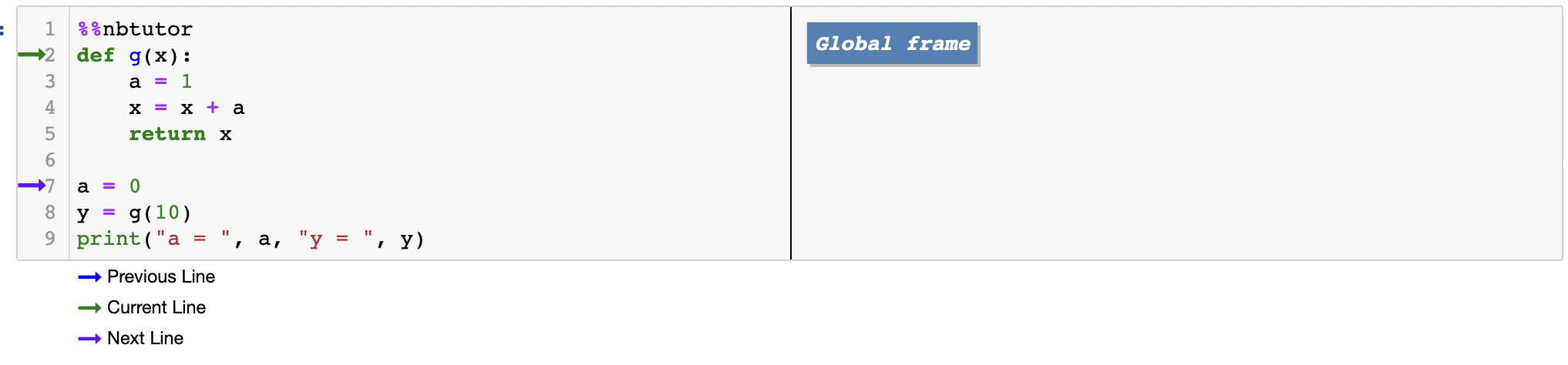• The function object is created, and g is bound to it within the global namespace.

• The name a is bound to 0, again in the global namespace.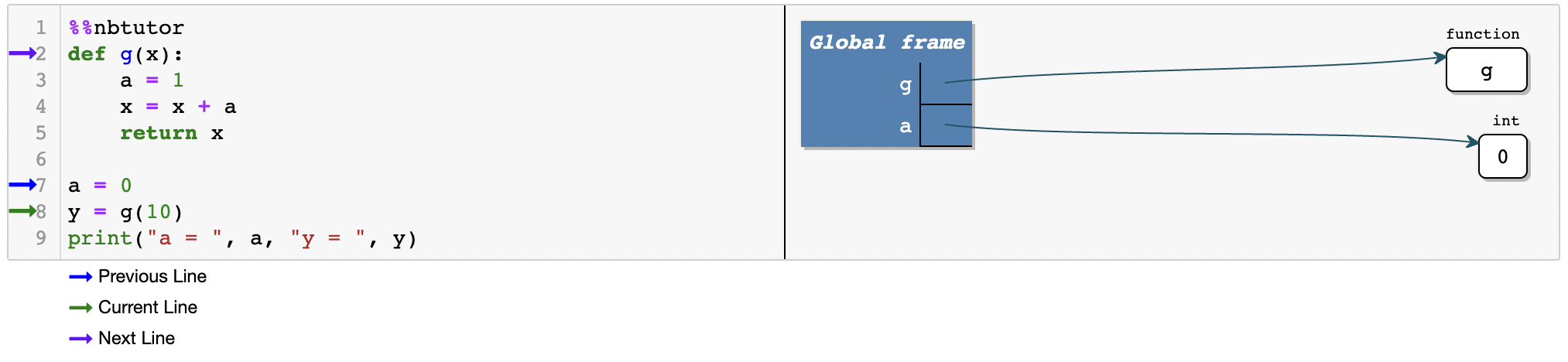Next g is called via y = g(10), leading to the following sequence of actions

• The local namespace for the function is created.

• Local names x and a are bound, so that the local namespace becomes {'x': 10, 'a': 1}.

• Note that the global a was not affected by the local a.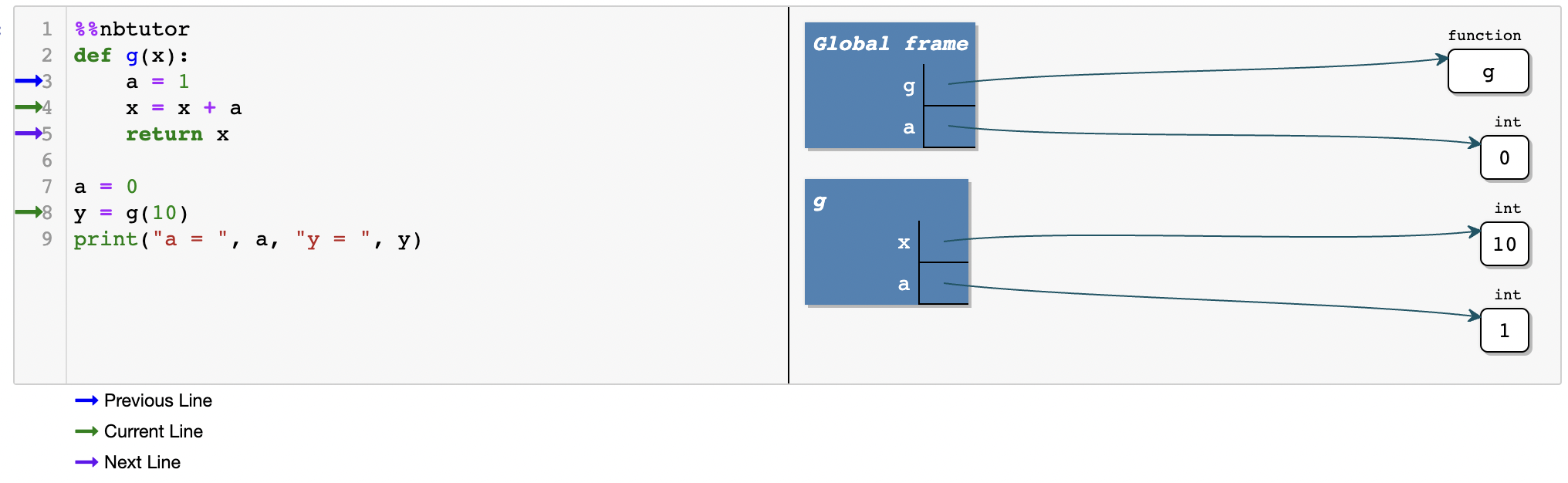• Statement x = x + a uses the local a and local x to compute x + a, and binds local name x to the result.

• This value is returned, and y is bound to it in the global namespace.

• Local x and a are discarded (and the local namespace is deallocated).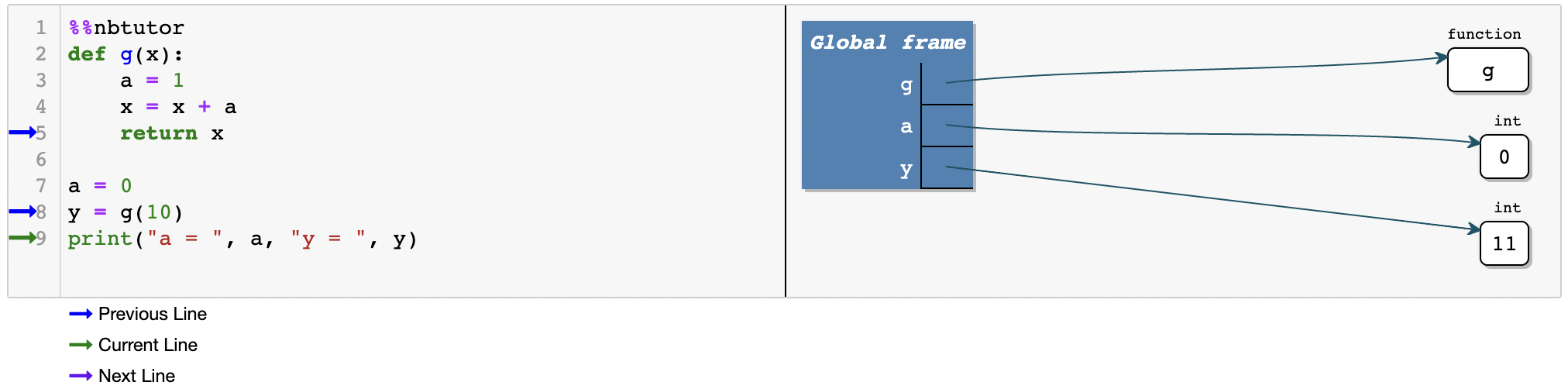### 6.3.9. Mutable Versus Immutable Parameters¶

This is a good time to say a little more about mutable vs immutable objects.

Consider the code segment

def f(x):
x = x + 1
return x

x = 1
print(f(x), x)

2 1


We now understand what will happen here: The code prints 2 as the value of f(x) and 1 as the value of x.

First f and x are registered in the global namespace.

The call f(x) creates a local namespace and adds x to it, bound to 1.

Next, this local x is rebound to the new integer object 2, and this value is returned.

None of this affects the global x.

However, it’s a different story when we use a mutable data type such as a list

def f(x):
x = x + 1
return x

x = 
print(f(x), x)

 


This prints  as the value of f(x) and same for x.

Here’s what happens

• f is registered as a function in the global namespace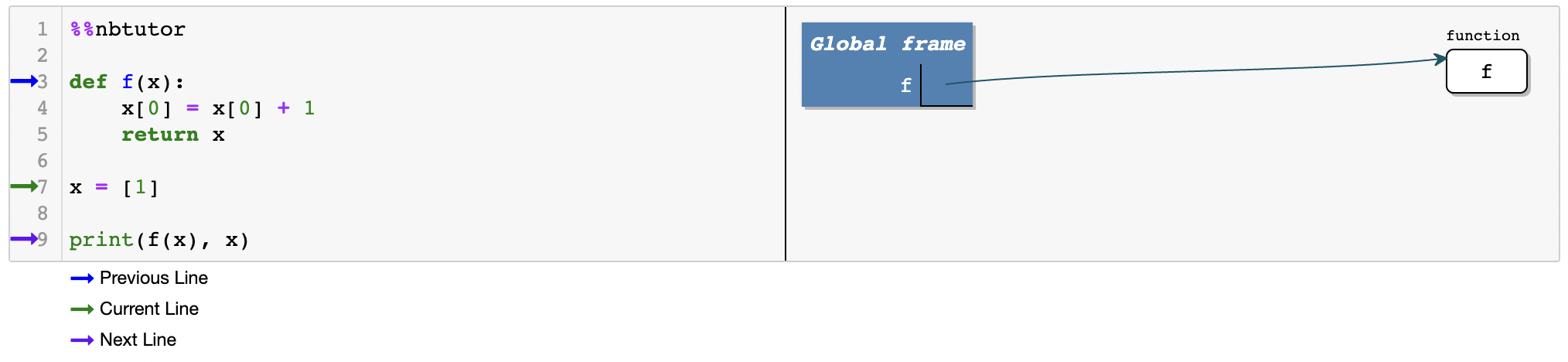• x bound to  in the global namespace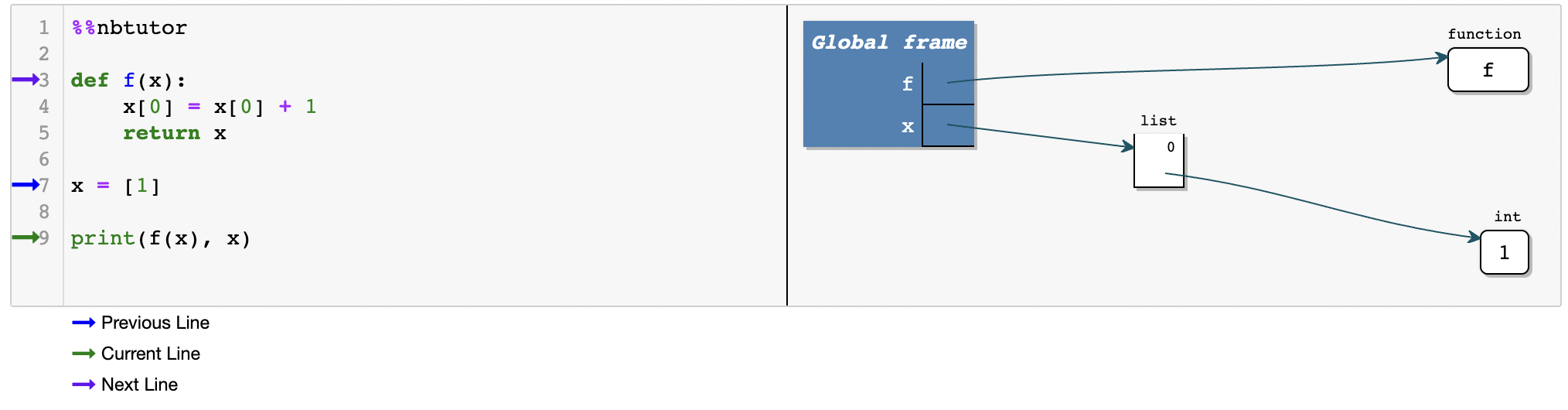• The call f(x)

• Creates a local namespace

• Adds x to the local namespace, bound to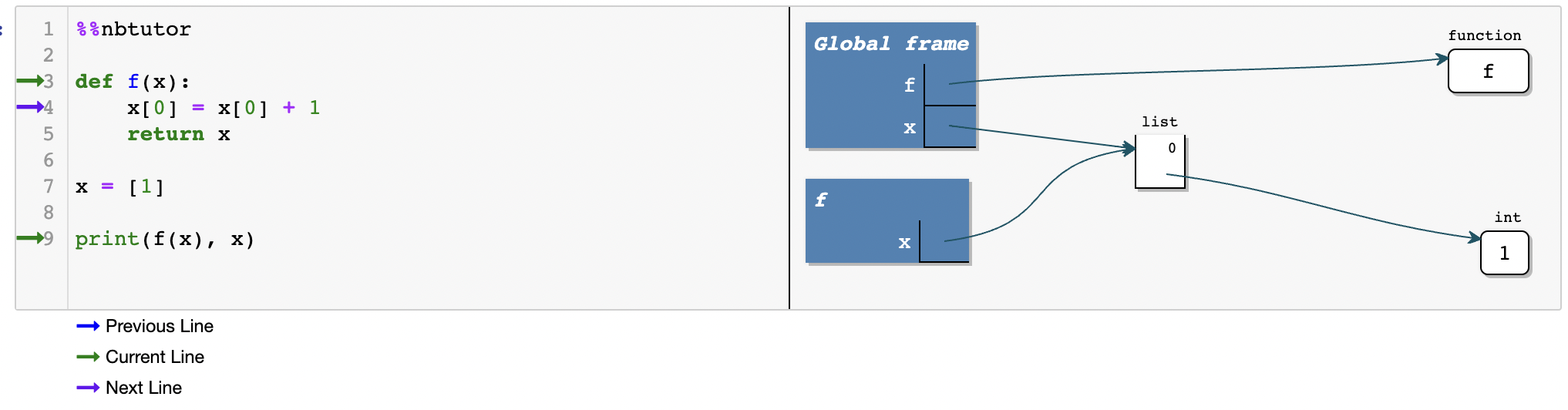Note

The global x and the local x refer to the same 

We can see the identity of local x and the identity of global x are the same

def f(x):
x = x + 1
print(f'the identity of local x is {id(x)}')
return x

x = 
print(f'the identity of global x is {id(x)}')
print(f(x), x)

the identity of global x is 139866415554048
the identity of local x is 139866415554048
 

• Within f(x)

• The list  is modified to 

• Returns the list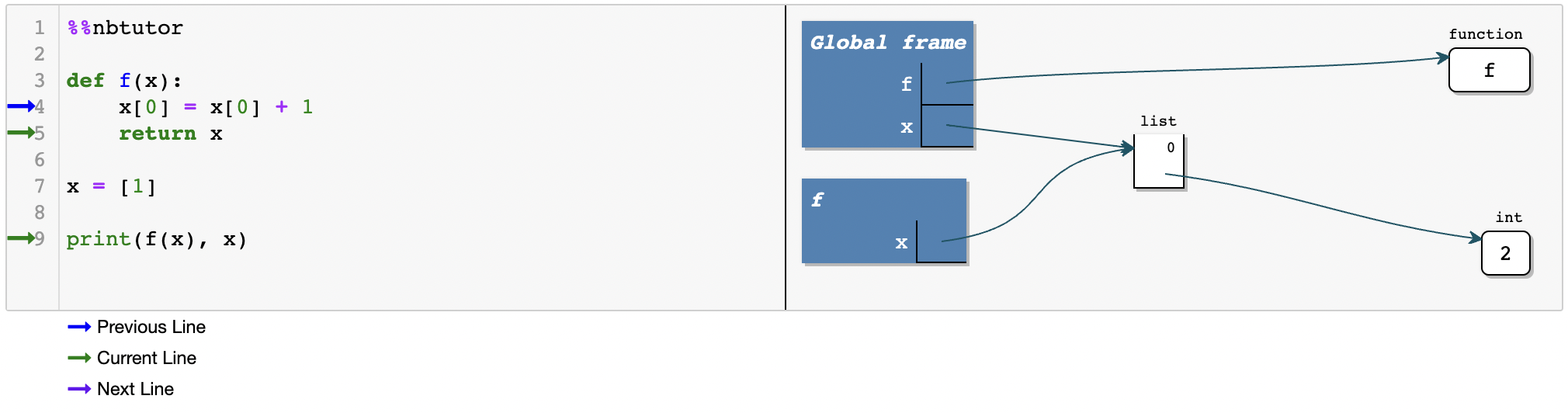• The local namespace is deallocated, and the local x is lost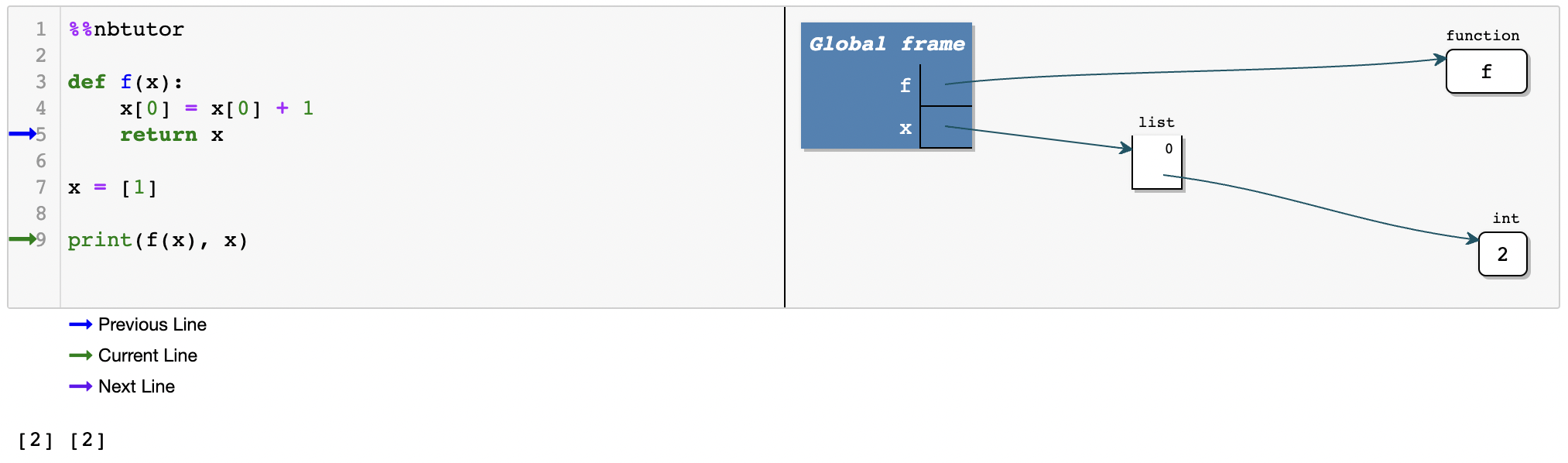If you want to modify the local x and the global x separately, you can create a copy of the list and assign the copy to the local x.

We will leave this for you to explore.

## 6.4. Summary¶

Messages in this lecture are clear:

• In Python, everything in memory is treated as an object.

• Zero, one or many names can be bound to a given object.

• Every name resides within a scope defined by its namespace.

This includes not just lists, strings, etc., but also less obvious things, such as

• functions (once they have been read into memory)

• modules (ditto)

• files opened for reading or writing

• integers, etc.

Consider, for example, functions.

When Python reads a function definition, it creates a function object and stores it in memory.

The following code illustrates further this idea

#reset the current namespace
%reset

def f(x): return x**2
f

<function __main__.f(x)>

type(f)

function

id(f)

139866422200784

f.__name__

'f'


We can see that f has type, identity, attributes and so on—just like any other object.

It also has methods.

One example is the __call__ method, which just evaluates the function

f.__call__(3)

9


Another is the __dir__ method, which returns a list of attributes.

We can also find f our current namespace

'f' in dir()

True


Modules loaded into memory are also treated as objects

import math

id(math)

139866501937808


We can find math in our global namespace after the import

print(dir()[-1::-1])

['quit', 'math', 'get_ipython', 'f', 'exit', '_oh', '_iii', '_ii', '_ih', '_i64', '_i63', '_i62', '_i61', '_i60', '_i59', '_i58', '_i57', '_i', '_dh', '__name__', '__builtins__', '__builtin__', '___', '__', '_63', '_62', '_61', '_60', '_59', '_58', '_57', '_', 'Out', 'In']


We can also find all objects associated with the math module in the private namespace of math

print(dir(math))

['__doc__', '__file__', '__loader__', '__name__', '__package__', '__spec__', 'acos', 'acosh', 'asin', 'asinh', 'atan', 'atan2', 'atanh', 'ceil', 'comb', 'copysign', 'cos', 'cosh', 'degrees', 'dist', 'e', 'erf', 'erfc', 'exp', 'expm1', 'fabs', 'factorial', 'floor', 'fmod', 'frexp', 'fsum', 'gamma', 'gcd', 'hypot', 'inf', 'isclose', 'isfinite', 'isinf', 'isnan', 'isqrt', 'lcm', 'ldexp', 'lgamma', 'log', 'log10', 'log1p', 'log2', 'modf', 'nan', 'nextafter', 'perm', 'pi', 'pow', 'prod', 'radians', 'remainder', 'sin', 'sinh', 'sqrt', 'tan', 'tanh', 'tau', 'trunc', 'ulp']


We can also directly import objects to our current namespace using from ... import ...

from math import log, pi, sqrt

print(dir()[-1::-1])

['sqrt', 'quit', 'pi', 'math', 'log', 'get_ipython', 'f', 'exit', '_oh', '_iii', '_ii', '_ih', '_i66', '_i65', '_i64', '_i63', '_i62', '_i61', '_i60', '_i59', '_i58', '_i57', '_i', '_dh', '__name__', '__builtins__', '__builtin__', '___', '__', '_63', '_62', '_61', '_60', '_59', '_58', '_57', '_', 'Out', 'In']


We can find these names appear in the current namespace now.

This uniform treatment of data in Python (everything is an object) helps keep the language simple and consistent.

## 6.5. Exercises¶

Exercise 6.1

We have met the boolean data type previously. Using what we have learnt in this lecture, print a list of methods of boolean objects.